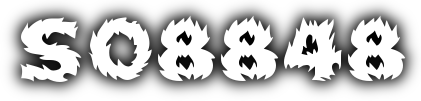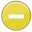#percent 中文解釋 wordnet sense Collocation Usage Collins Definition
Noun
/pərˈsent/,Font size:By a specified amount in or for every hundred,
1. One part in every hundred
• - a reduction of half a percent or so in price
2. The rate, number, or amount in each hundred; percentage
• - the percent of drug users who are infected
1. By a specified amount in or for every hundred
• - new car sales may be down nineteen percent
• - staff rejected a 1.8 percent increase

1. percentage: a proportion in relation to a whole (which is usually the amount per hundred)
2. In mathematics, a percentage is a way of expressing a number as a fraction of 100 (per cent meaning "per hundred" in Latin). It is often denoted using the percent sign, "%", or the abbreviation "pct". For example, 45% (read as "forty-five percent") is equal to 45/100, or 0.45.
3. A part or other object per hundred; percentage
4. a ratio where the second term is 100.
5. Ratio of the item's frequency to the number of all records.
6. per hundred, one part in 100. Commonly used for describing increases or decreases over time. If the price of an item has increased from £10 to £11, it is said to have risen by 10%.
7. a rate expressed in hundredths between two values with same UoM (a type of numeric)
8. One part in a 100. A percentage of a number is found by dividing it by the base figure. If the question is what percentage is 36 to 72, then 72 is the base and the divisor. 36 divided by 72 = 50 or 50%.
9. A given part of a whole, meaning that 100% of something is all of it. 50% of something is half of that whole.
10. A ratio or fraction in which the second term on denominator is always 100.
11. Based on the whole amount, divided into 100 parts. Each of the 100 parts is one percent.
12. Enter percentage. Split percentages should add up to 100%. Fraction is allowed up to 2 decimal places, e.g. 33.33%.
13. 21 CFR 201.10: The term, if used without qualifications, means percent on a weight-in-weight basis.
14. Always use numerals and spell out percent.
15. One word. Spell out in continuous text. Use symbol in tables, figures, and charts. Use the shortest pronounceable form eg 20–30% not 20%–30%
16. A special ratio that compares a number to 100 using the symbol %.
17. Stands for hundredths. Example: 4% is 4 parts of one hundred, or 4/100
18. Fractions with a denominator of 100. The percentage is the numerator of the fraction, indicating the number of parts out of 100.
19. a number of parts out of 100 of something. For example, a quarter (25 cents) is 25 percent of a dollar (100 cents).
20. percent by weight, used usually for lead-based paint (1 percent = 10,000 μg/gram.
21. Dimmer levels are expressed as a percentage of the supply voltage. (100% = 230 – 240 Volts(UK)).
22. Represents a percentage used as a basis to calculate an element